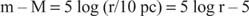## Absolute Magnitudes

To use the magnitude system to compare stars on an absolute scale means removal of the distance effect. An absolute magnitude (for which astronomers use the symbol M) is defined as the apparent magnitude a star would have if it were placed at a standard distance of 10 parsecs (32.6 light‐years). It can be shown that the difference between apparent and absolute magnitude is related to the distance aswhere distance r is expressed in parsecs. This difference m – M is called the distance modulus. m – M = –5 corresponds to a distance r = 1 pc, m – M = 0 corresponds to r = 10 pc, m – M = +5 corresponds to r = 100 pc, and so on. If the Sun were moved to a distance of 10 pc, it would appear as a 5th magnitude star, that is, M ∼ +5 (it is useful to remember this, that a star of 1 solar luminosity corresponds to an absolute magnitude of +5).